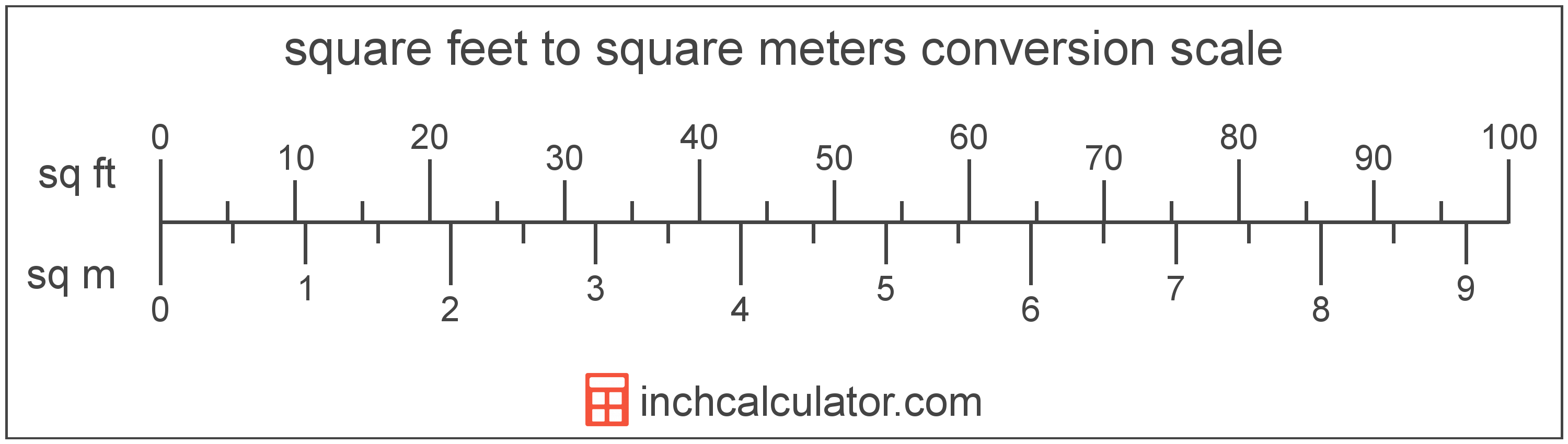# Square Feet to Square Meters Converter

Enter the area in square feet below to get the value converted to square meters.

(find square feet)
Results in Square Meters:1 sq ft = 0.092903 sq m

Do you want to convert square meters to square feet?

## How to Convert Square Feet to Square Meters

To convert a measurement in square feet to a measurement in square meters, multiply the area by the following conversion ratio: 0.092903 square meters/square foot.

Since one square foot is equal to 0.092903 square meters, you can use this simple formula to convert:

square meters = square feet × 0.092903

The area in square meters is equal to the area in square feet multiplied by 0.092903.

For example, here's how to convert 5 square feet to square meters using the formula above.
square meters = (5 sq ft × 0.092903) = 0.464515 sq m## What Is a Square Foot?

One square foot is equivalent to the area of a square with sides that are each 1 foot in length. One square foot is equal to 144 square inches or 0.092903 square meters .

The square foot is a US customary and imperial unit of area. A square foot is sometimes also referred to as a square ft. Square feet can be abbreviated as sq ft, and are also sometimes abbreviated as ft². For example, 1 square foot can be written as 1 sq ft or 1 ft².

You can use a square footage calculator to calculate the area of a space if you know its dimensions.

## What Is a Square Meter?

One square meter is equivalent to the area of a square with sides that are each 1 meter in length. One square meter is equal to 10.76391 square feet or 10,000 square centimeters.

The square meter, or square metre, is the SI derived unit for area in the metric system. A square meter is sometimes also referred to as a square m. Square meters can be abbreviated as sq m, and are also sometimes abbreviated as . For example, 1 square meter can be written as 1 sq m or 1 m².

You can use a square meters calculator to calculate the area of a space if you know its dimensions.

## Square Foot to Square Meter Conversion Table

Table showing various square foot measurements converted to square meters.
Square Feet Square Meters
1 sq ft 0.092903 sq m
2 sq ft 0.185806 sq m
3 sq ft 0.278709 sq m
4 sq ft 0.371612 sq m
5 sq ft 0.464515 sq m
6 sq ft 0.557418 sq m
7 sq ft 0.650321 sq m
8 sq ft 0.743224 sq m
9 sq ft 0.836127 sq m
10 sq ft 0.92903 sq m
11 sq ft 1.0219 sq m
12 sq ft 1.1148 sq m
13 sq ft 1.2077 sq m
14 sq ft 1.3006 sq m
15 sq ft 1.3935 sq m
16 sq ft 1.4864 sq m
17 sq ft 1.5794 sq m
18 sq ft 1.6723 sq m
19 sq ft 1.7652 sq m
20 sq ft 1.8581 sq m
21 sq ft 1.951 sq m
22 sq ft 2.0439 sq m
23 sq ft 2.1368 sq m
24 sq ft 2.2297 sq m
25 sq ft 2.3226 sq m
26 sq ft 2.4155 sq m
27 sq ft 2.5084 sq m
28 sq ft 2.6013 sq m
29 sq ft 2.6942 sq m
30 sq ft 2.7871 sq m
31 sq ft 2.88 sq m
32 sq ft 2.9729 sq m
33 sq ft 3.0658 sq m
34 sq ft 3.1587 sq m
35 sq ft 3.2516 sq m
36 sq ft 3.3445 sq m
37 sq ft 3.4374 sq m
38 sq ft 3.5303 sq m
39 sq ft 3.6232 sq m
40 sq ft 3.7161 sq m

## References

1. Merriam-Webster, square foot, https://www.merriam-webster.com/dictionary/square%20foot
2. Collins Dictionary, Definition of 'square meter', https://www.collinsdictionary.com/us/dictionary/english/square-meter